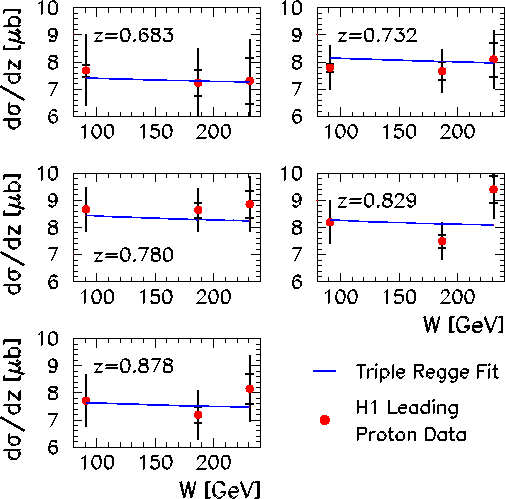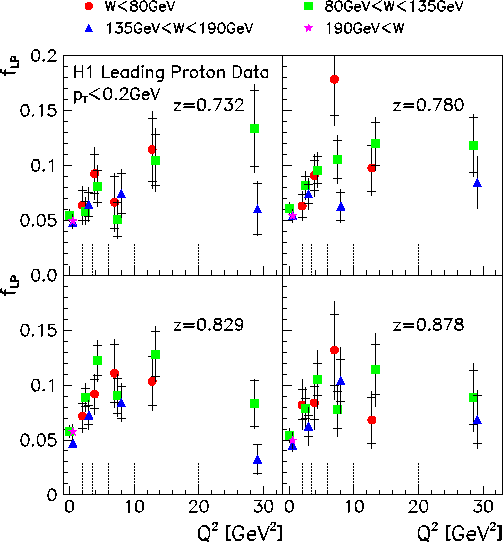# Photoproduction with a Leading Proton at HERA

Diffractive interactions, i.e. those in which the scattering only takes place peripherally, have been of interest to experimentalists and theorists for a long time. They have been studied both in hadron hadron and in lepton hadron collisions. These processes are characterized by a small momentum transfer, resulting in small scattering angles. The scattering partners can each either stay intact or dissociate. In a Regge motivated picture, the interaction is thought of as a t-channel exchange of particles. Depending on the kinematic range, the type of the exchange varies.

At HERA, both real and virtual photons, emitted by a beam electron, can collide with a beam proton. The first is referred to as photoproduction, the latter as deep inelastic scattering (DIS). The photon and the proton may undergo the hadronic interaction mentioned. We distinguish cases where the elastically scattered proton retains almost all of its momentum (z=1) from those where it loses a substantial fraction (here: 10-30%, such that 0.7<z<0.9). This paper completes a set of investigations in the following way:
Photoproduction DIS
z=1  Z.Phys.C74(1997)221 Z.Phys.C6(1997)613
z<1  this paper Eur.Phys.J.C6(1999)587-602

### The Process gamma p -> Xp'

We focus on the reaction gamma p -> X p' for real photons. The process is sketched in the figure. The data sample comprises events where a leading proton was detected under small scattering angles using the H1 forward proton spectrometer some 80 m away from the main detector. The photon was required to be real by requiring the electron to be found in one of the small angle electron detectors, such that the photon virtuality is small (Q2<0.01GeV2). The differential cross-section dsigma(W,z)/dz was measured in the kinematic range W=91-231 GeV, z=0.66-0.90 and pt(p')<0.2GeV.

### Kinematic Dependence of the Production RateThe differential cross section dsigma(W,z)/dz for gamma p -> X p' is shown in the adjacent figure. z varies between 0.878 and 0.683, which implies that the proton lost a longitudinal momentum of 100 to 260 GeV in the collision with the photon.

The production rate is found to be independent of W and z within experimental errors. The average value is 8.05 +/- 0.06(stat) +/- 0.89(syst) microbarn.

The effective composition of the exchanges hence exhibit only a soft W and z dependence over the range studied.

Quantitatively a fit based on a Regge inspired model gives a good representation of the data. In this model, the process is thought of as the exchange of an effective trajectory alphai between the photon and the proton. The total cross section for the interaction between alphai and the photon is modelled by the exchange of a further effective trajectory alphak. The fit results are the intercepts of the trajectories, alphai(0)=0.33 +/- 0.04(stat) +/- 0.04(syst) and alphak(0)=0.99 +/- 0.01(stat) +/- 0.05(syst), as well as an indirectly determined value for the slope parameter b describing the t dependence according to ebt, b=6.6 +/- 0.7(stat) +/- 1.5(syst) GeV-2 at z=0.78. This confirms previous findings that within the z range under study, reactions involving a pomeron are suppressed relative to those with pions and Reggeons. Additionally, the result for alphak is consistent with the scattering between the photon and alphai being mediated by Pomeron exchange, which is the dominant contribution at the center of mass energies of the alphai-photon system reached here (32GeV < MX < 130GeV).### From Real to Virtual Photons

Based on the diagram above the fraction of all events that contain leading protons should not depend on the photon  virtuality Q2. The photon component ought to factorize.

The validity of this simple picture has been investigated. The result is depicted in the adjacent figure. The fraction of all events per unit z that contain a leading proton, fLP, has been studied as a function of Q2 to investigate the transition from real to virtual photons in bins of W for four values of z. Surprisingly this fraction rises with Q2.